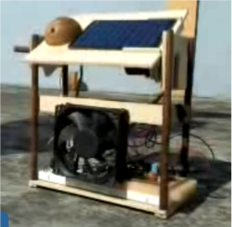# Simple solar traker with ARDUINOThe solar tracker made ​​with a control board low-cost Arduino using recycled materials.

A project focused on a quick and easy construction taking into account the subsequent reuse of all the elements used.

The constant control of a servo motor based on a series of photo-sensitive sensors determine the behavior of the system, the photocell powers a rechargeable 9-volt battery that supplies the energy necessary to activate the servo motor and an additional fan installed to check feeding of a photo cell.

The flat surface that supports the photo cell is automatically aligned at 90 degrees to the major source of light detected and follows the course of this in either directions of rotation of the motor. When the light source (the sun) is lost in the west the system automatically returns to its rest position to the east ready to receive the rising sun the following morning.

The following code was used in Arduino:

 int v1 = 35; if (valor3 < 700){ } int v2 = 0; a = 1; if (a==1){ int analog = 0; } v1 = v1 – 1; int p= 0; } } int servo = 2; } if (a==3){ int valor1 = 0; if (valor2 > 700) { v1 = v1 + 1; int valor2 = 0; if (valor1 < 700){ } int valor3 = 0; if (valor3 < 700){ if (a==4){ int a = 0; a = 2; v1 = 35; void setup() } } { } if (v1>0){ pinMode(servo, OUTPUT); } if (v1<180){ Serial.begin(9600); if (valor3 > 700) { } pinMode(1, INPUT); if (valor2 < 700){ else{ pinMode(2, INPUT); if (valor1 < 700){ v1 = 180; pinMode(3, INPUT); a = 3; } } } } void loop() } else{ { } v1 = 0; valor1 = analogRead(1); if (valor3 < 700) { } valor2 = analogRead(2); if (valor2 < 700){ v2 = map(v1,0,180,500,2500); valor3 = analogRead(3); if (valor1 < 700){ digitalWrite(servo, HIGH); a = 2; a = 4; delayMicroseconds(v2); if (valor1 > 700) { } digitalWrite(servo, LOW); if (valor2 < 700){ } }